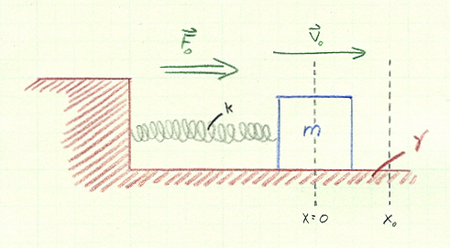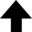I am ashamed to tell you to how many figures I carried these computations, having no other business at the time.Isaac Newton, after computing the natural logarithm of $1 + x$ to more than 50 decimal places

How can we determine the period of a pendulum that is not already in motion? The obvious way is to disturb the system and observe the resulting behavior. It can be found that the response of a system to perturbation can tell us something about the nature of that same system in the absence of a disturbance. As such, this illustration is an extension of The Simple Pendulum.

## Motion of a Driven Damped Linear Oscillator

This program solves the equation of motion of a driven, damped linear oscillator to illustrate how a harmonic system responds to perturbation.
$\displaystyle{ \sum F_x = m a_x }$ $\displaystyle{ A_0 \cos (\omega t) - k x - \gamma \frac{\mathrm{d} x}{\mathrm{d} t} = m \frac{\mathrm{d}^2 x}{\mathrm{d} t^2} }$Initial position $x_0$ of the mass: m0.0 ≤ $x_0$ ≤ 1.0 m Initial velocity $v_0$ of the mass: m/sec0.0 ≤ $v_0$ ≤ 6.0 m/sec Value of $k/m$: Value of the damping coefficient $\gamma$: kg/sec0.0 ≤ $\gamma$ ≤ 1.0 kg/sec Amplitude of the driving force $A_0$: N0.0 ≤ $A_0$ ≤ 5.0 N Frequency of the driving force $\omega$: Hz0.0 ≤ $\omega$ ≤ 7.5 Hz Time-step $\mathrm{d} t$ of the system: sec0.001 ≤ $\mathrm{d} t$ ≤ 0.1 sec Steps: Total simulation time: sec
0%
Current data point:

Legend: Position (m); Velocity (m/sec); Acceleration (m/sec2)